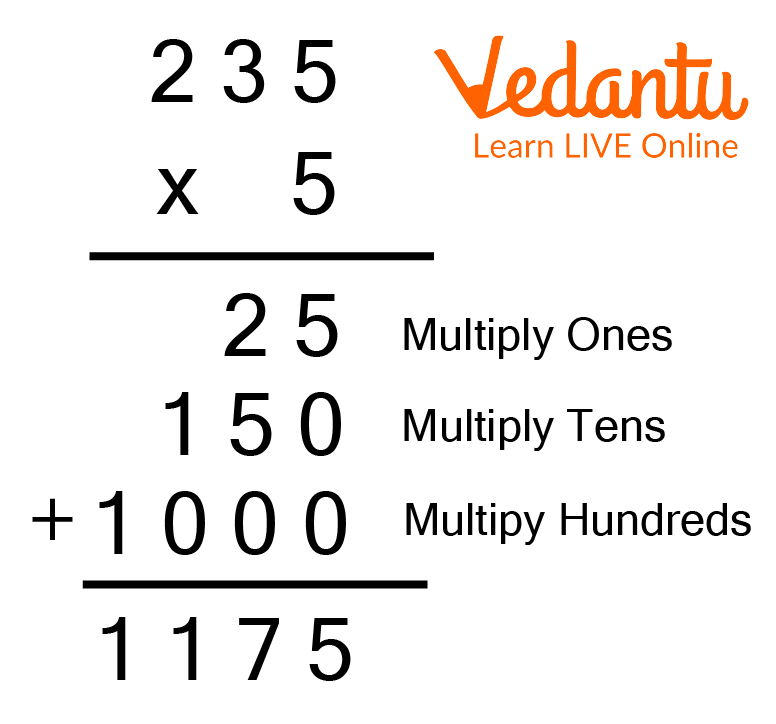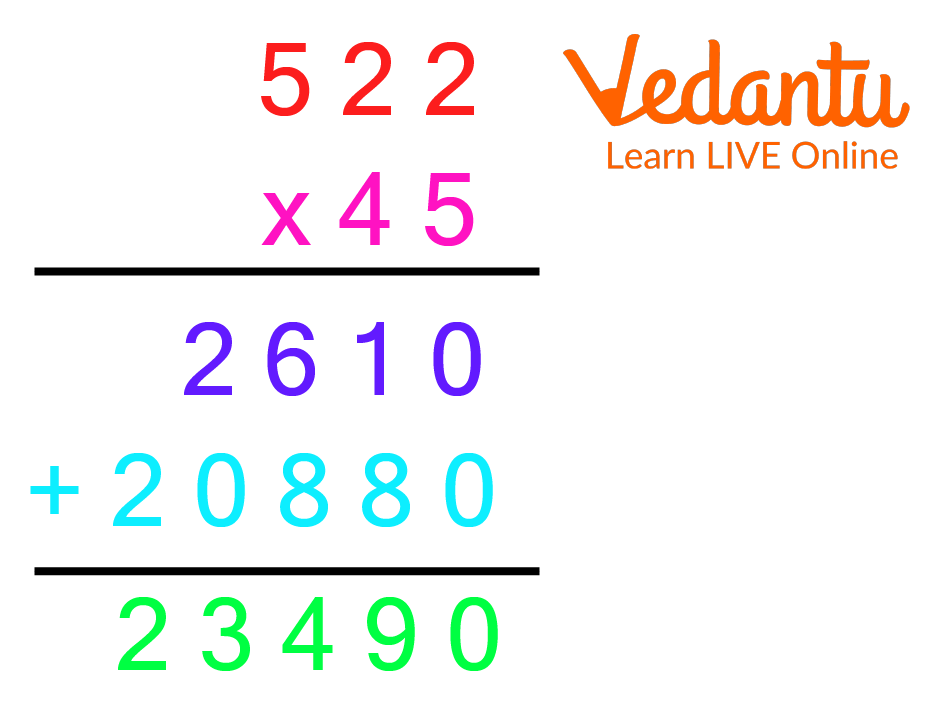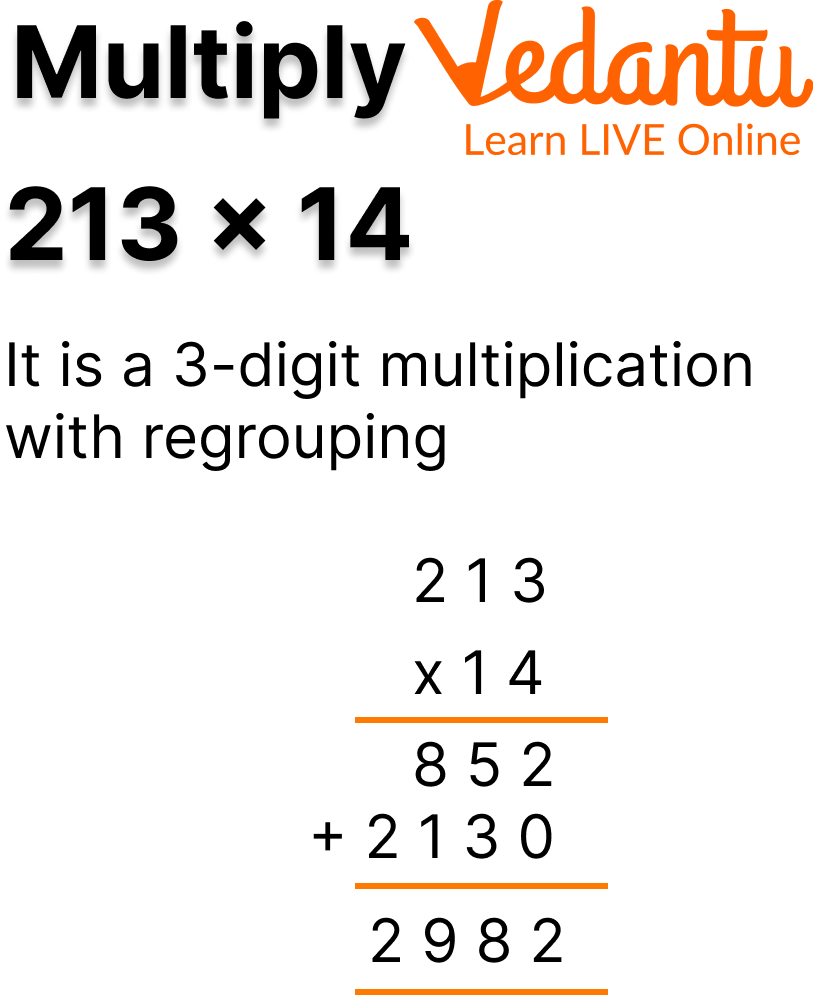Courses
Courses for Kids
Free study material
Free LIVE classes
More

# 3-Digit MultiplicationLIVE
Join Vedantu’s FREE Mastercalss

## Introduction to 3-Digit Multiplication

3-digit multiplication involves multiplying 3-digit numbers with 1-digit, 2-digit, and 3-digit numbers by placing them at their correct place values. This multiplication goes difficult with 2 and 3-digit numbers. However, multiplying 3-digit with 1-digit is quite simple.

Therefore, in this article, we will learn about 3-digit with 1-digit multiplication, 3-digit with 2-digit multiplication, and 3-digit with 3-digit multiplication along with regrouping and without regrouping numbers. We will understand these through detailed steps and solve examples so you can have a good grasp of concepts and solve questions easily.3-Digit Multiplication with 1-Digit

## What is 3-Digit Multiplication?

It is a method of multiplying 3-digit numbers with other numbers. During the multiplication, the numbers are arranged in the columns as per their place values. The 3-digit numbers are arranged according to their place values as ones, tens, and hundreds.

While multiplying the two sets of digits, the larger number is kept on the top and the smaller number is placed below it. The number placed on top is called the multiplicand and the number placed below is called the multiplier.An Example of 3-Digit Multiplication

After arranging the numbers according to their place values, the multiplier is multiplied by all the digits of the multiplicand one by one starting from the left. Firstly, the one digit is multiplied, followed by the tens digit, and then the hundreds digit. Thus, the product obtained is the final result.

Let us understand this with some multiplication examples.

Example 1 – When we multiply 211 by 3, we get 633.Multiplication of 211 by 3

Let us now understand how to do this multiplication with other numbers.

## Multiplication of a 3-Digit By a 1-Digit Number

In this type of multiplication, there are two cases:

• 3-Digit Multiplication without Regrouping In this multiplication, the 1-digit number is simply multiplied by the 3-digit number without any carry-overs.

For example –Multiply 314×2Multiplication of 314 by 2

• 3-Digit Multiplication with Regrouping It is multiplication in which the 3-digit number is multiplied by the 1-digit number using the carry-overs.

For example – Multiply 315×2Multiplication of 315 by 2

## Multiplication of a 3-Digit By a 2-Digit Number

In this type of multiplication (3-digit), we multiply the digits by placing the 3-digit on the top and the 2-digit number below it. This multiplication is also performed with regrouping and without regrouping.An Example of 3-Digit Multiplication with 2-Digit

During the multiplication of a 3-digit number by a 2-digit number, the one digit of the multiplier is multiplied with the multiplicand. Then, zero is placed under the one digit of the number obtained. After this, the tens digit of the multiplier is multiplied with the multiplicand. Then, both these products are added to get the final result.

Let us discuss this through examples.

Example 1 – Multiply 511×21Multiplication of 511 by 21

Example 2 – Multiply 213×14

It is a 3-digit multiplication with regroupingMultiplication of 213 by 14

## Multiplication of a 3-Digit By a 3-Digit Number

Before doing multiplication, arrange the numbers according to their place values. Firstly, the one digit of the multiplier is multiplied by the multiplicand, followed by the tens digit, and then the hundreds digit.

The products so obtained will get added to obtain the final product. The method of this multiplication is the same as the previous one.

Let us discuss some multiplication sums with answers to understand this.

Example 1 – Multiply 311×123Multiply 311 by 123

Here two zeroes are placed under ones and tens digit.

Example 2 – Multiply 314×213Multiply 314 by 213

## Summary

3-digit multiplication is multiplication in which each digit of the multiplier is multiplied with the multiplicand. This multiplication is of three types. These are:

• 3-digitby1-digit multiplication

• 3-digit by 2-digit multiplication

• 3-digit by 3-digit multiplication

The above multiplication types have two scenarios, with regrouping and without regrouping. The 3-digit multiplication becomes easy if 3-digit multiplication tricks are followed correctly.

Last updated date: 27th Sep 2023
Total views: 116.1k
Views today: 4.16k

## FAQs on 3-Digit Multiplication

1. What are the properties of multiplication?

The properties of multiplication are:

• Commutative Property – It states that there will be no change in the result if the order of multiplication is changed.

Example: 3×4 = 4×3 = 12

• Associative Property – It states that the order of multiplication doesn't matter if we multiply three or more numbers.

Example: (2×4)×6 = 2×(4×6) = 48

• Distributive Property – It states that if a number is multiplied by the sum of two digits, the result will be equal to the sum of products obtained by multiplying that number with those two numbers individually.

Example: 3×(2+2) = (3×2)+(3×2) = 12

2. Why are 3-digit multiplications important?

By learning 3-digit multiplication, children will get to know basic mathematical concepts and will be able to perform complex tasks. They can solve questions related to division, fractions, and algebra. Their problem-solving skills will get developed. The concepts of 3-digit multiplication help students understand how the question will be solved. They can implement this knowledge for various applications such as calculating the cost of the same items, evaluating sales tax, finding areas, etc. That is why the fundamentals of 3-digit multiplication are very important.

3. What is multiplication?

The fundamental concept of repeatedly adding the same number is represented by the process of multiplication. The results of multiplying two or more numbers are known as the product of those numbers, and the factors that are multiplied are referred to as the factors. The process of combining matrices, vectors, or other quantities under specific rules to obtain their product.